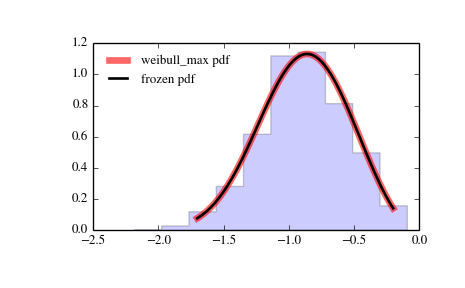scipy.stats.weibull_max¶

scipy.stats.weibull_max = <scipy.stats._continuous_distns.frechet_l_gen object at 0x2b45d2fca750>[source]

A Frechet left (or Weibull maximum) continuous random variable.

Continuous random variables are defined from a standard form and may require some shape parameters to complete its specification. Any optional keyword parameters can be passed to the methods of the RV object as given below:

Parameters: x : array_like quantiles q : array_like lower or upper tail probability c : array_like shape parameters loc : array_like, optional location parameter (default=0) scale : array_like, optional scale parameter (default=1) size : int or tuple of ints, optional shape of random variates (default computed from input arguments ) moments : str, optional composed of letters [‘mvsk’] specifying which moments to compute where ‘m’ = mean, ‘v’ = variance, ‘s’ = (Fisher’s) skew and ‘k’ = (Fisher’s) kurtosis. (default=’mv’) Alternatively, the object may be called (as a function) to fix the shape, location, and scale parameters returning a “frozen” continuous RV object: rv = weibull_max(c, loc=0, scale=1) Frozen RV object with the same methods but holding the given shape, location, and scale fixed.

weibull_max
The same distribution as frechet_l.

Notes

The probability density function for frechet_l is:

frechet_l.pdf(x, c) = c * (-x)**(c-1) * exp(-(-x)**c)

for x < 0, c > 0.

Examples

>>> from scipy.stats import weibull_max
>>> import matplotlib.pyplot as plt
>>> fig, ax = plt.subplots(1, 1)

Calculate a few first moments:

>>> c = 2.86879617091
>>> mean, var, skew, kurt = weibull_max.stats(c, moments='mvsk')

Display the probability density function (pdf):

>>> x = np.linspace(weibull_max.ppf(0.01, c),
...               weibull_max.ppf(0.99, c), 100)
>>> ax.plot(x, weibull_max.pdf(x, c),
...          'r-', lw=5, alpha=0.6, label='weibull_max pdf')

Alternatively, freeze the distribution and display the frozen pdf:

>>> rv = weibull_max(c)
>>> ax.plot(x, rv.pdf(x), 'k-', lw=2, label='frozen pdf')

Check accuracy of cdf and ppf:

>>> vals = weibull_max.ppf([0.001, 0.5, 0.999], c)
>>> np.allclose([0.001, 0.5, 0.999], weibull_max.cdf(vals, c))
True

Generate random numbers:

>>> r = weibull_max.rvs(c, size=1000)

And compare the histogram:

>>> ax.hist(r, normed=True, histtype='stepfilled', alpha=0.2)
>>> ax.legend(loc='best', frameon=False)
>>> plt.show()Methods

 rvs(c, loc=0, scale=1, size=1) Random variates. pdf(x, c, loc=0, scale=1) Probability density function. logpdf(x, c, loc=0, scale=1) Log of the probability density function. cdf(x, c, loc=0, scale=1) Cumulative density function. logcdf(x, c, loc=0, scale=1) Log of the cumulative density function. sf(x, c, loc=0, scale=1) Survival function (1-cdf — sometimes more accurate). logsf(x, c, loc=0, scale=1) Log of the survival function. ppf(q, c, loc=0, scale=1) Percent point function (inverse of cdf — percentiles). isf(q, c, loc=0, scale=1) Inverse survival function (inverse of sf). moment(n, c, loc=0, scale=1) Non-central moment of order n stats(c, loc=0, scale=1, moments=’mv’) Mean(‘m’), variance(‘v’), skew(‘s’), and/or kurtosis(‘k’). entropy(c, loc=0, scale=1) (Differential) entropy of the RV. fit(data, c, loc=0, scale=1) Parameter estimates for generic data. expect(func, c, loc=0, scale=1, lb=None, ub=None, conditional=False, **kwds) Expected value of a function (of one argument) with respect to the distribution. median(c, loc=0, scale=1) Median of the distribution. mean(c, loc=0, scale=1) Mean of the distribution. var(c, loc=0, scale=1) Variance of the distribution. std(c, loc=0, scale=1) Standard deviation of the distribution. interval(alpha, c, loc=0, scale=1) Endpoints of the range that contains alpha percent of the distribution

Previous topic

scipy.stats.weibull_min

Next topic

scipy.stats.wrapcauchy